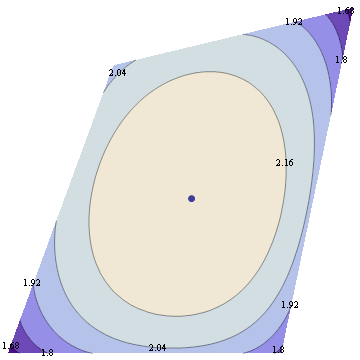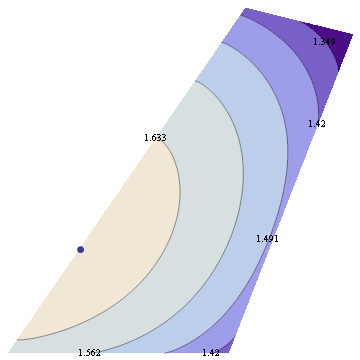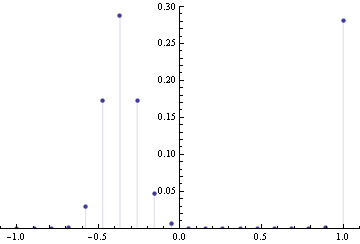## Thursday, January 17, 2008

### Maximum entropy with skewness constraint

Maximum entropy principle is the idea that we should should pick a distribution maximizing entropy subject to certain constraints. Many known distributions come out in this way, such as Gamma, Poisson, Normal, Beta, Exponential, Geometric, Cauchy, log-normal, and others. In fact, there's a close connection between maxent distributions and exponential families -- Darmois-Koopman-Pitman theorem promises that all positive distributions with finite sufficient statistics correspond to exponential families. Recall that exponential families are families that are log-linear in the space of parameters, so in a sense, exponential families are analogous to vector spaces -- whereas vector space is closed under addition, exponential family is closed under multiplication.

After you pick the form of your constraints, the maxent distribution is determined by the value of the constraints. So in this sense, constraint values serve as sufficient statistics, hence by DKP theorem, result must lie in the exponential family determined by form of the constraints.

You can use Lagrange multipliers (see my previous post) to maximize entropy subject to moment constraints. This works to get Normal distribution and Exponential, but if you introduce skewness constraints you get distribution of the form exp(ax+bx^2+cx^3)/Z, which fails to normalize unless c=0, so what went wrong?

This is due to a technical point which doesn't matter for deriving most distributions -- correspondence between maxent and exponential family distributions only holds for distributions that are strictly positive. Also, entropy maximization subject to moment constraints is valid only if non-negativity constraints are inactive. In certain cases, like this one, you must also include nonnegativity constraints, and then the solution fails to have a nice closed form.

Suppose we maximize entropy in a distribution with 6 bins in the interval [-1,1] with constraints that mean=0, variance=0.3 and skewness=0. In addition to normalization constraint, this leaves a 2 dimensional space of valid distributions, and we can plot the space of valid (nonnegative) distributions, along with their entropy.Now suppose we change skewness constraint to be 0.15 The new plot is below.You can see that the maximum entropy solution lies right on the edge of the space of valid distributions, hence the nonnegativity constraint must be active at this point.

To get a sense what the maxent solution might look like, we can discretize the distribution, and do constrained optimization to find a solution. Below is the solution for 20 bins, variance=0.4 and skewness=0.23I tried various values of positive skewness, and that seems to be a general form -- distribution is 0 for positive x's except for a single spike at largest allowable x, for negative x's there's a Gaussian-like hump.

References:

-- Mathematica notebook, web version
-- Justification of MaxEnt from statistical point of view by Tishby/TichochinskyAnonymous said...

Hi!

Im a grad student learning ML for the first time and Im really stuck in a problem.

Im designing a program to detect "what is fashion" in a certain place. For this, I have collected data in this place and have read thru several appraoches (decision trees, candidate elimination etc). However, my data has only "yes" values ie Ive only got postive instances whereas it looks like these algorithms require both positive and negative instances.
Im really stuck up in this problem and I have nowhere to turn to. Can u please suggest an approach or tell me where Ive gone wrong?
Thanks
Shanker

Yaroslav Bulatov said...

I don't really understand the description, please specify:

1. What type of training data your learner gets (attribute types, etc)
2. What kind of task the learner is expected to execute (what input, what output)Anonymous said...

The question is "What is the fashion in secondlife (the online game)". For this purpose, I logged on to second life and collected data of several people I encountered(about 150 data sets).

1. So the attributes are
Gender - Male/Female
Hair - Bald/Short/Long/Hat
Top - Fullsleeve/Halfsleeve/Tanktop
Topcolor - Black/Brown/Blue

So the different data sets I collected have different combinations of these. I have to divide this into training and testing data.

2. Output : Well, the question is what clothes are fashionable, so i expect it has to tell me Yes/No for any testing data.

My problem : I have no negative training instances (examples of 'Not Fashion'). Is it still possible to make a program?
I have learnt Decision trees/candidate elimination/naive bayes. I also have Tom mitchell book.

Yaroslav Bulatov said...

So you only have examples of fashionable and no examples of unfashionable? It sounds like an unsupervised learning problem. One approach is to learn a distribution over your training data, then test how well it fits test data. IE, take a set of feature functions {f_i}(y)={isFemale(y),isShort(y),...} then fit a distribution of the form p(y)=exp(w.f(y))/Z(w) where w is your vector of adjustable weights. Z(w) is a quantity needed to make your quantity normalize.

For this approach you will need to learn how to fit log-linear models using maximum likelihood to data. Actually you could treat this probability distribution as a conditional random field where "x" variable is the same for every datapoint. There are some good CRF tutorials out there, including a recent one by Sutton/McCallum

Unknown said...

Hey, I see this is an old post, but did you even find the correct answer? You have some very good insights but your answer is not correct. The correct answer is a Gaussian with the desired mean and variance, plus small distant spike. Say the spike is second Gaussian, centered at z, with variance 1/z and weight w*z^-3. In the limit of z->Inf, this spike's contribution to the mean, variance, and entropy of the distribution will vanish, but it will contribute finite w to the skew. Since this pdf already maximizes entropy given the mean/variance constraints, it also maximizes entropy given a third constraint. So that you observed in your simulation wasn't f[x] trying to go to zero; a full minimization algorithm would have pushed your spike out to infinite while fleshing the main mass out into a Gaussian.

But if you are still examining problems like this, please get in touch, because I've been working on these tricky Var Calc issues for a while now.

Unknown said...

I mean did you EVER find the correct answer. Sorry.

bollings said...

If you do things with the skewness then you will know about the slop of that things and let you know about the quality thigns too. http://www.dissertationdataanalysis.com/comprehensive-help-with-data-analysis-in-research/help-with-data-analysis-in-qualitative-research/ is the best one place to know about the quality writing rules.

Unknown said...

I think things like this are really interesting. I absolutely love to find unique places like this. It really looks super creepy though!! Best Machine Learning institute in Chennai | Best Machine learning training | best machine learning training certification

draj said...

Excellent machine learning blog,thanks for sharing...
Seo Internship in Bangalore
Smo Internship in Bangalore
Digital Marketing Internship Program in Bangalore

john said...
john said...